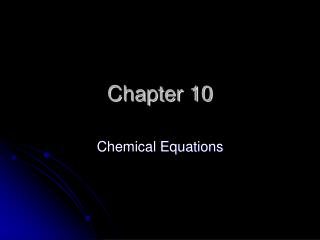# Chapter 10 - PowerPoint PPT PresentationDownload PresentationChapter 10

Chapter 10Download Presentation## Chapter 10

- - - - - - - - - - - - - - - - - - - - - - - - - - - E N D - - - - - - - - - - - - - - - - - - - - - - - - - - -
##### Presentation Transcript

1. Chapter 10 Chemical Equations

2. A chemical reaction is a reaction in which at least one new substance is formed. • Example – Hydrogen and oxygen react to form water.

3. A chemical equation is a shorthand method of writing a chemical reaction using chemical symbols and formulas. • Example – H2 + O2  H2O

4. Symbols Used in Equations • (g) – gas • (cr) – crystalline solid • (l) – liquid • (aq) – aqueous solution

5. Law of Conservation of Mass • In an ordinary chemical reaction, mass can neither be created nor destroyed. • Mass of products must equal the mass of the reactants.

6. Balancing Chemical Equations • 1. Count the number of atoms of each element on both sides of the equation. • 2.Use coefficients to balance the equation. • 3. Always use the lowest possible ratio of coefficients to balance.

7. Examples

8. Practice Website • www.chem.vt.edu/RVGS/ACT/notes/scripts/bal_eq1.html

9. Balancing NO-NO’s • Coefficients can only be added BEFORE a chemical symbol or formula. • NEVER change a subscript to balance an equation.

10. Signs a Chemical Reaction Has Occurred: • Color change • Liberation of a gas • Energy change • Formation of a precipitate • Change in odor

11. 5 Major Types of Chemical Reactions 1. Synthesis Reaction – 2 or more reactants combine to form one product. Example-

12. Rust is the product of a synthesis reaction.

13. 2. Decomposition Reaction – the breakdown of one reactant into its components. Example -

14. 3. Single Replacement Reaction – one element replaces another in a compound. Example -

15. 4. Double Replacement Reaction – the positive and negative ions of two reactants exchange places. Example-

16. Many times a precipitate is the result of a double replacement reaction.

17. 5. Combustion – the burning of an organic compound in oxygen to form CO2 and H2O and energy. Example -

18. Welding is an example of a combustion reaction.

19. Practice – Classifying Reactions • Cl2 + 2KBr  KCl + Br2 • 2Ag2O  4Ag + O2 • 2Na + Cl2  2NaCl • PbCl2 + Li2SO4  PbSO4 + 2LiCl

20. Activote Quiz CaO + H2O  Ca(OH)2 This equation is an example of which type of chemical reaction: • Decomposition • Synthesis • Combustion • Single Replacement

21. LAB – Chemical Reactions

22. Writing Chemical Equations 7 Diatomic elements Chlorine Fluorine Iodine Bromine Oxygen Nitrogen Hydrogen

23. Common Acids • Sulfuric acid • Nitric acid • Carbonic acid • Hydrochloric acid • Acetic acid • Phosphoric acid